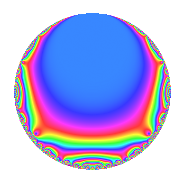# Properties

 Label 4018.2.a.baLevel 4018 Weight 2 Character orbit 4018.a Self dual Yes Analytic conductor 32.084 Analytic rank 0 Dimension 2 CM No Inner twists 1

# Related objects

## Newspace parameters

 Level: $$N$$ = $$4018 = 2 \cdot 7^{2} \cdot 41$$ Weight: $$k$$ = $$2$$ Character orbit: $$[\chi]$$ = 4018.a (trivial)

## Newform invariants

 Self dual: Yes Analytic conductor: $$32.0838915322$$ Analytic rank: $$0$$ Dimension: $$2$$ Coefficient field: $$\Q(\sqrt{2})$$ Coefficient ring: $$\Z[a_1, a_2, a_3]$$ Coefficient ring index: $$1$$ Fricke sign: $$-1$$ Sato-Tate group: $\mathrm{SU}(2)$

## $q$-expansion

Coefficients of the $$q$$-expansion are expressed in terms of $$\beta = \sqrt{2}$$. We also show the integral $$q$$-expansion of the trace form.

 $$f(q)$$ $$=$$ $$q$$ $$+ q^{2}$$ $$+ \beta q^{3}$$ $$+ q^{4}$$ $$-2 \beta q^{5}$$ $$+ \beta q^{6}$$ $$+ q^{8}$$ $$- q^{9}$$ $$+O(q^{10})$$ $$q$$ $$+ q^{2}$$ $$+ \beta q^{3}$$ $$+ q^{4}$$ $$-2 \beta q^{5}$$ $$+ \beta q^{6}$$ $$+ q^{8}$$ $$- q^{9}$$ $$-2 \beta q^{10}$$ $$-3 \beta q^{11}$$ $$+ \beta q^{12}$$ $$-4 q^{15}$$ $$+ q^{16}$$ $$+ ( -2 + 4 \beta ) q^{17}$$ $$- q^{18}$$ $$+ ( 4 - \beta ) q^{19}$$ $$-2 \beta q^{20}$$ $$-3 \beta q^{22}$$ $$+ ( 4 + 2 \beta ) q^{23}$$ $$+ \beta q^{24}$$ $$+ 3 q^{25}$$ $$-4 \beta q^{27}$$ $$+ ( 4 + 4 \beta ) q^{29}$$ $$-4 q^{30}$$ $$+ ( 4 + 2 \beta ) q^{31}$$ $$+ q^{32}$$ $$-6 q^{33}$$ $$+ ( -2 + 4 \beta ) q^{34}$$ $$- q^{36}$$ $$-6 \beta q^{37}$$ $$+ ( 4 - \beta ) q^{38}$$ $$-2 \beta q^{40}$$ $$+ q^{41}$$ $$+ ( 4 + 4 \beta ) q^{43}$$ $$-3 \beta q^{44}$$ $$+ 2 \beta q^{45}$$ $$+ ( 4 + 2 \beta ) q^{46}$$ $$+ ( 2 - 5 \beta ) q^{47}$$ $$+ \beta q^{48}$$ $$+ 3 q^{50}$$ $$+ ( 8 - 2 \beta ) q^{51}$$ $$+ 12 q^{53}$$ $$-4 \beta q^{54}$$ $$+ 12 q^{55}$$ $$+ ( -2 + 4 \beta ) q^{57}$$ $$+ ( 4 + 4 \beta ) q^{58}$$ $$+ ( 4 + 2 \beta ) q^{59}$$ $$-4 q^{60}$$ $$-6 q^{61}$$ $$+ ( 4 + 2 \beta ) q^{62}$$ $$+ q^{64}$$ $$-6 q^{66}$$ $$+ ( -4 + 3 \beta ) q^{67}$$ $$+ ( -2 + 4 \beta ) q^{68}$$ $$+ ( 4 + 4 \beta ) q^{69}$$ $$+ ( -2 - \beta ) q^{71}$$ $$- q^{72}$$ $$+ ( 8 - 4 \beta ) q^{73}$$ $$-6 \beta q^{74}$$ $$+ 3 \beta q^{75}$$ $$+ ( 4 - \beta ) q^{76}$$ $$+ ( -6 + 3 \beta ) q^{79}$$ $$-2 \beta q^{80}$$ $$-5 q^{81}$$ $$+ q^{82}$$ $$+ ( -12 + 4 \beta ) q^{83}$$ $$+ ( -16 + 4 \beta ) q^{85}$$ $$+ ( 4 + 4 \beta ) q^{86}$$ $$+ ( 8 + 4 \beta ) q^{87}$$ $$-3 \beta q^{88}$$ $$+ ( 6 - 4 \beta ) q^{89}$$ $$+ 2 \beta q^{90}$$ $$+ ( 4 + 2 \beta ) q^{92}$$ $$+ ( 4 + 4 \beta ) q^{93}$$ $$+ ( 2 - 5 \beta ) q^{94}$$ $$+ ( 4 - 8 \beta ) q^{95}$$ $$+ \beta q^{96}$$ $$+ ( 2 + 4 \beta ) q^{97}$$ $$+ 3 \beta q^{99}$$ $$+O(q^{100})$$ $$\operatorname{Tr}(f)(q)$$ $$=$$ $$2q$$ $$\mathstrut +\mathstrut 2q^{2}$$ $$\mathstrut +\mathstrut 2q^{4}$$ $$\mathstrut +\mathstrut 2q^{8}$$ $$\mathstrut -\mathstrut 2q^{9}$$ $$\mathstrut +\mathstrut O(q^{10})$$ $$2q$$ $$\mathstrut +\mathstrut 2q^{2}$$ $$\mathstrut +\mathstrut 2q^{4}$$ $$\mathstrut +\mathstrut 2q^{8}$$ $$\mathstrut -\mathstrut 2q^{9}$$ $$\mathstrut -\mathstrut 8q^{15}$$ $$\mathstrut +\mathstrut 2q^{16}$$ $$\mathstrut -\mathstrut 4q^{17}$$ $$\mathstrut -\mathstrut 2q^{18}$$ $$\mathstrut +\mathstrut 8q^{19}$$ $$\mathstrut +\mathstrut 8q^{23}$$ $$\mathstrut +\mathstrut 6q^{25}$$ $$\mathstrut +\mathstrut 8q^{29}$$ $$\mathstrut -\mathstrut 8q^{30}$$ $$\mathstrut +\mathstrut 8q^{31}$$ $$\mathstrut +\mathstrut 2q^{32}$$ $$\mathstrut -\mathstrut 12q^{33}$$ $$\mathstrut -\mathstrut 4q^{34}$$ $$\mathstrut -\mathstrut 2q^{36}$$ $$\mathstrut +\mathstrut 8q^{38}$$ $$\mathstrut +\mathstrut 2q^{41}$$ $$\mathstrut +\mathstrut 8q^{43}$$ $$\mathstrut +\mathstrut 8q^{46}$$ $$\mathstrut +\mathstrut 4q^{47}$$ $$\mathstrut +\mathstrut 6q^{50}$$ $$\mathstrut +\mathstrut 16q^{51}$$ $$\mathstrut +\mathstrut 24q^{53}$$ $$\mathstrut +\mathstrut 24q^{55}$$ $$\mathstrut -\mathstrut 4q^{57}$$ $$\mathstrut +\mathstrut 8q^{58}$$ $$\mathstrut +\mathstrut 8q^{59}$$ $$\mathstrut -\mathstrut 8q^{60}$$ $$\mathstrut -\mathstrut 12q^{61}$$ $$\mathstrut +\mathstrut 8q^{62}$$ $$\mathstrut +\mathstrut 2q^{64}$$ $$\mathstrut -\mathstrut 12q^{66}$$ $$\mathstrut -\mathstrut 8q^{67}$$ $$\mathstrut -\mathstrut 4q^{68}$$ $$\mathstrut +\mathstrut 8q^{69}$$ $$\mathstrut -\mathstrut 4q^{71}$$ $$\mathstrut -\mathstrut 2q^{72}$$ $$\mathstrut +\mathstrut 16q^{73}$$ $$\mathstrut +\mathstrut 8q^{76}$$ $$\mathstrut -\mathstrut 12q^{79}$$ $$\mathstrut -\mathstrut 10q^{81}$$ $$\mathstrut +\mathstrut 2q^{82}$$ $$\mathstrut -\mathstrut 24q^{83}$$ $$\mathstrut -\mathstrut 32q^{85}$$ $$\mathstrut +\mathstrut 8q^{86}$$ $$\mathstrut +\mathstrut 16q^{87}$$ $$\mathstrut +\mathstrut 12q^{89}$$ $$\mathstrut +\mathstrut 8q^{92}$$ $$\mathstrut +\mathstrut 8q^{93}$$ $$\mathstrut +\mathstrut 4q^{94}$$ $$\mathstrut +\mathstrut 8q^{95}$$ $$\mathstrut +\mathstrut 4q^{97}$$ $$\mathstrut +\mathstrut O(q^{100})$$

## Embeddings

For each embedding $$\iota_m$$ of the coefficient field, the values $$\iota_m(a_n)$$ are shown below.

For more information on an embedded modular form you can click on its label.

Label $$\iota_m(\nu)$$ $$a_{2}$$ $$a_{3}$$ $$a_{4}$$ $$a_{5}$$ $$a_{6}$$ $$a_{7}$$ $$a_{8}$$ $$a_{9}$$ $$a_{10}$$
1.1
 −1.41421 1.41421
1.00000 −1.41421 1.00000 2.82843 −1.41421 0 1.00000 −1.00000 2.82843
1.2 1.00000 1.41421 1.00000 −2.82843 1.41421 0 1.00000 −1.00000 −2.82843
 $$n$$: e.g. 2-40 or 990-1000 Significant digits: Format: Complex embeddings Normalized embeddings Satake parameters Satake angles

## Inner twists

This newform does not admit any (nontrivial) inner twists.

## Atkin-Lehner signs

$$p$$ Sign
$$2$$ $$-1$$
$$7$$ $$-1$$
$$41$$ $$-1$$

## Hecke kernels

This newform can be constructed as the intersection of the kernels of the following linear operators acting on $$S_{2}^{\mathrm{new}}(\Gamma_0(4018))$$:

 $$T_{3}^{2}$$ $$\mathstrut -\mathstrut 2$$ $$T_{5}^{2}$$ $$\mathstrut -\mathstrut 8$$ $$T_{11}^{2}$$ $$\mathstrut -\mathstrut 18$$